# 晶体学的基本概念

## 晶体和点阵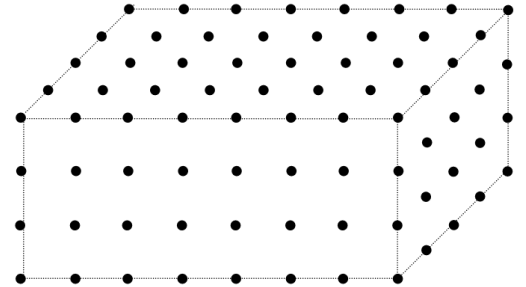## 晶胞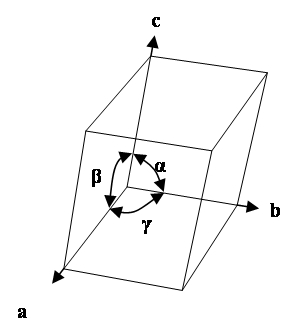## 布拉维点阵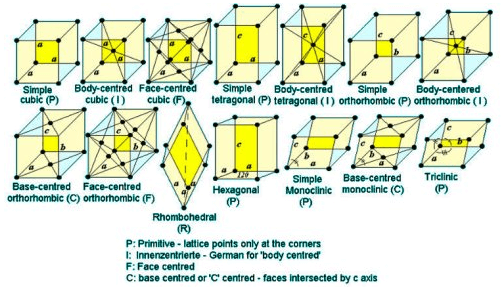## 基元## 晶体对称性

### 点群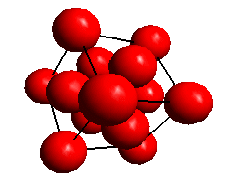### 晶系

 System Symmetry Element Conventional Unit Cell Triclinic One fold axis a ≠ b ≠ c; α ≠ β ≠ γ Monoclinic Two fold axis (diad) a ≠ b ≠ c; α = γ = 90°, β > 90° Orthorhombic Three mutually perpendicular diads a ≠ b ≠ c; α = β = γ = 90° Trigonal Three fold axis (triad) a = b = c; α = β = γ < 120°, ≠ 90° Tetragonal Four fold axis (tetrad) a = b ≠ c; α = β = γ = 90° Hexagonal Six fold axis (hexad) a = b ≠ c; α = β = 90°, γ = 120 ° Cubic Four triads (parallel to <111>) a = b = c; α = β = γ = 90°
Adapted from "Essentials of Crystallography" McKie D and McKie C, Blackwell Scientific Publications (1986)

### 布拉维点阵

 System Lattice Symbol Conventional Unit Cell Number of lattice points Triclinic P a ≠ b ≠ c; α ≠ β ≠ γ 1 Monoclinic P a ≠ b ≠ c; α = γ = 90°, β > 90° 1 Monoclinic C a ≠ b ≠ c; α = γ = 90°, β > 90° 2 Orthorhombic P a ≠ b ≠ c; α = β = γ = 90° 1 Orthorhombic C a ≠ b ≠ c; α = β = γ = 90° 2 Orthorhombic I a ≠ b ≠ c; α = β = γ = 90° 2 Orthorhombic F a ≠ b ≠ c; α = β = γ = 90° 4 Tetragonal P a = b ≠ c; α = β = γ = 90° 1 Tetragonal I a = b ≠ c; α = β = γ = 90° 2 Cubic P a = b = c; α = β = γ = 90° 1 Cubic I a = b = c; α = β = γ = 90° 2 Cubic F a = b = c; α = β = γ = 90° 4 Trigonal R a = b ≠ c; α = β = 90°, γ = 120° or         a = b = c; α = β = γ < 120°, ≠ 90° 3 Hexagonal P a = b ≠ c; α = β = 90°, γ = 120° 1
Adapted from "Essentials of Crystallography" McKie D and McKie C, Blackwell Scientific Publications (1986)The fourteen Bravais lattices

### 劳埃群

 Crystal System Laue ID Symmetry Example Phases Triclinic -1 1 Inversion centre as the only symmetry Plagioclase Feldspar (e.g. NaAlSi₃O₈) Monoclinic 2/m 2 Two-fold rotation axis perpendicular to mirror plane Clinopyroxenes (e.g. Ca(Mg,Fe)Si₂O₆); Monoclinic ZrO₂ Orthorhombic mmm 3 Three perpendicular mirror planes Olivine ((Mg,Fe)₂SiO₄); Cementite (Fe₃C) Tetragonal 4/m 4 Four-fold axis with one perpendicular mirror plane Leucite (KAlSi₂O₆) Tetragonal 4/mmm 5 Four-fold axis with three perpendicular mirror planes Rutile (TiO₂); γ-TiAl Trigonal -3 6 Three-fold rotation-inversion Ilmenite (FeTiO₃) Trigonal -3m 7 Three-fold rotation-inversion with mirror plane Quartz (SiO₂), α-alumina (Al₂O₃) Hexagonal 6/m 8 Six-fold axis with perpendicular mirror Apatite (Ca₅(PO₄)₃(OH,F,Cl)) Hexagonal 6/mmm 9 Six-fold axis with three perpendicular mirror planes Magnesium, α-Titanium Cubic m3 10 Three-fold axis with one mirror Pyrite (FeS₂) Cubic m3m 11 Three-fold axis with two mirror planes Fe-BCC; Fe-FCC; Aluminium
Table showing the 11 Laue classes, along with some typical example phases.

## 晶向、镜面和晶带轴

### 晶向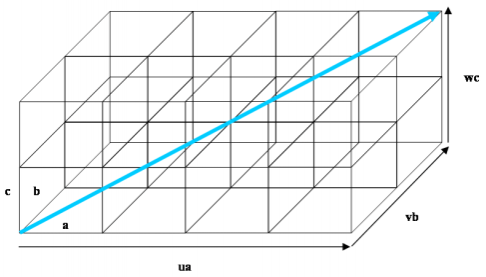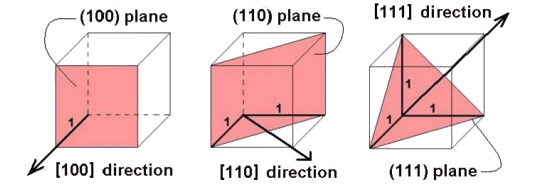### 晶面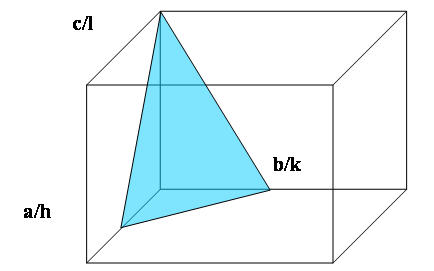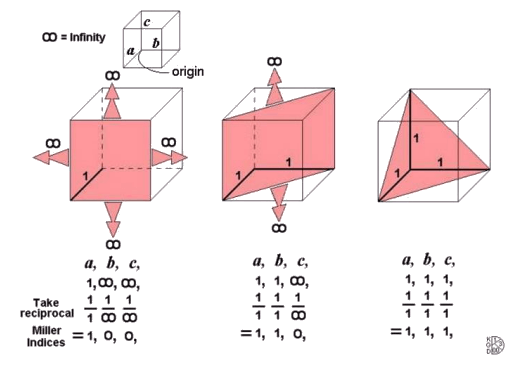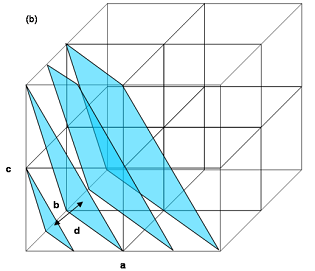### 晶带轴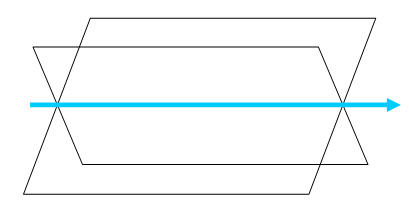u=(2U-V)/3

v=(2V-U)/3

t=-(U+V)/3

w=W